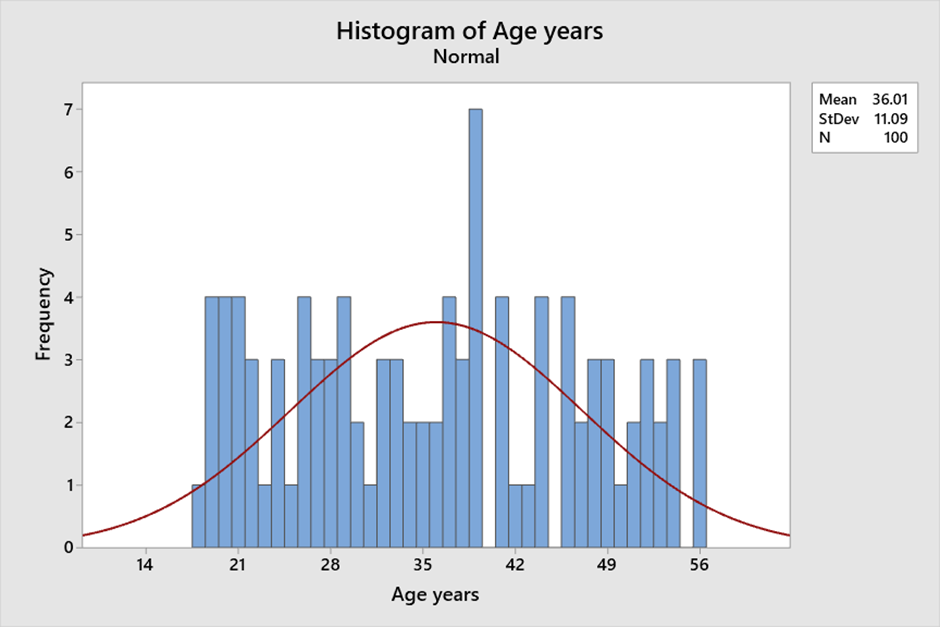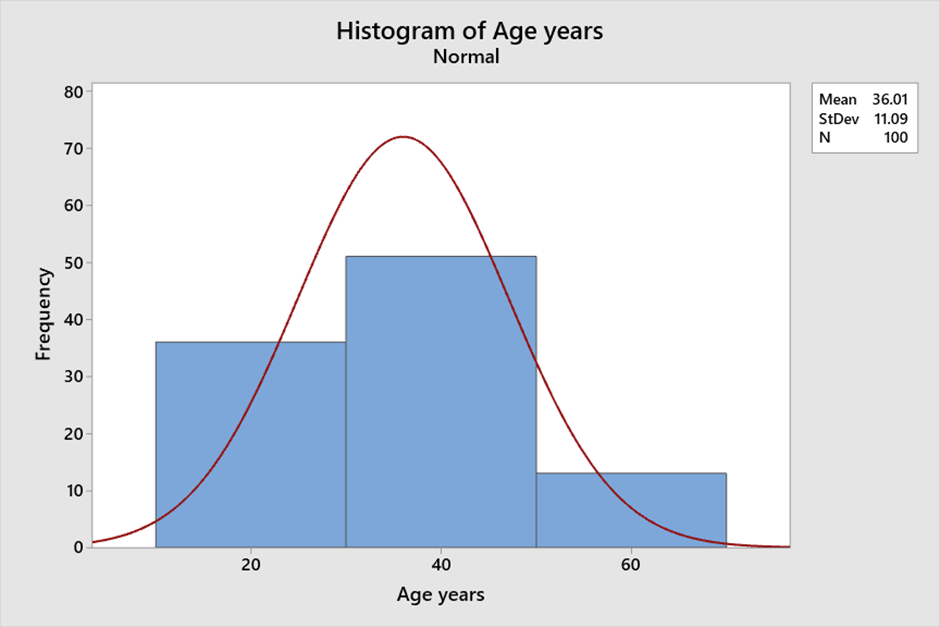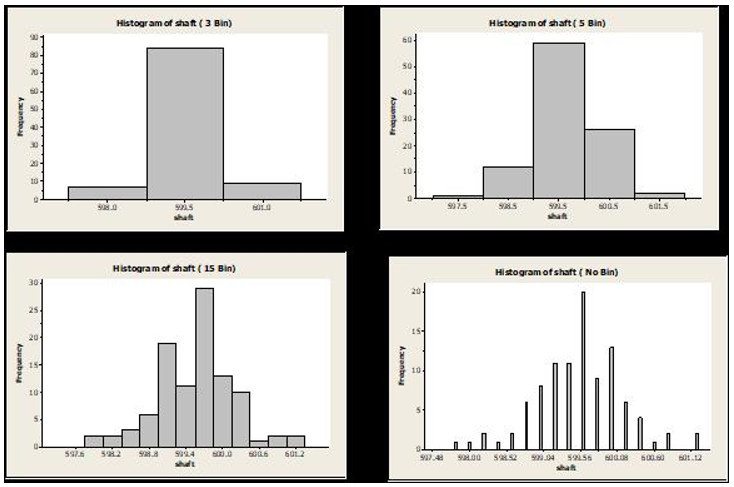• 0

# Histogram

Histogram (from the Greek histos meaning mast of the ship – vertical bars of the histogram) of a sample of numerical values is a plot which involves rectangles which represent frequency of occurrence in a specific interval. A Histogram can be used to assess the central tendency, shape and spread of continuous sample data.

An application-oriented question on the topic along with responses can be seen below. The best answer was provided by Partho Karmakar on 12th May 2023.

Applause for all the respondents - Amit Kumar Shukla, Raghavendra Rao Althar, Partho Karmakar, Ramjanam Singh.

## Question

Q 563. Histogram is a common visualization tool for continuous data. With examples explain how histogram can help in understanding the central tendency, variability and shape of the distribution?? How does selecting a bin size affect the understanding on these 3 things?

Note for website visitors -

## Recommended Posts

• 0

A histogram is a graphical representation of numerical data that provides insight into the distribution of the data. It is created by grouping the data into bins and then plotting the frequency of each bin as a bar. This visual representation of the data allows for easy interpretation of the central tendency, variability, and shape of the data. Histograms are a useful tool for visualizing and understanding the data and can help in data analysis and decision-making.

Central tendency: The central tendency of a distribution refers to the value around which the data tends to cluster. A histogram can help to identify the central tendency of the data by showing the peak of the distribution. The peak of the distribution corresponds to the most frequently occurring value. For example, consider the following data set of ages (in years) of a group of 100 people: 18 to 56 . We can see that there is a peak around age 40 and that the distribution is roughly symmetric.

Variability: The spread of data is called variability. A histogram can help to identify the variability of the data by showing the width of the distribution. A wider distribution indicates greater variability, while a narrower distribution indicates less variability. For example, consider the following data set of Age (in years) of a group of 100 people: 18 - 56. If we create a histogram with bins of width 1,  there is not a lot of variability in the heights of the group.

Shape: The shape of the distribution refers to the overall pattern of the data. Histograms help identify the shape of the data by displaying its symmetry or skewness. For example, consider the following same data set of Age (in years) of a group of 100 people: 18 – 56. If we create a histogram with bins of width 20, we can see that the distribution is roughly symmetric, indicating ages are evenly distributed around the mean.

Bin size: The bin size refers to the width of each bin in the histogram. The bin size can affect the central tendency, variability, and shape of the distribution. If the bin size is too small, the histogram will have too many bars, making it difficult to interpret. If the bin size is too large, the histogram will have too few bars, potentially obscuring important features of the data.

Example:

Consider the following data set of ages (in years) of a group of 100 people: 18 to 56 with different bin sizes. If we create a histogram with bins of width 1, we can see that the distribution is relatively uniform and that there are no clear peaks or valleys. However, if we increase the bin size to 20, we can see that there is a peak around age 40 and that the distribution is roughly symmetric.

With Bin Size 1:With Bin size 20:In summary, histograms can provide insight into the central tendency, variability, and shape of the data. The bin size can affect these features, so it is important to choose an appropriate bin size that accurately reflects the characteristics of the data.

##### Share on other sites

• 0

A histogram displays numerical data by grouping data into "bins" of equal width. Each bin is plotted as a bar whose height corresponds to how many data points are in that bin. Bins are also sometimes called "intervals", "classes", or "buckets"

A histogram shows x-axis a define interval (particular bin). The height of each bar (y-axis) represents the number of count - in the data set that fall within a particular bin.

Central tendency: Central tendency is that value which represents the characteristics of the entire dataset considering each and every value in the set of data. The three measures of central tendency are Mean, Median, and Mode

Variability: Variability means the tendency to shift or change — of being "variable."

Shape of the distribution: The distribution shape of - data can be define  logical order to the values, and the 'low' and 'high' end values on the x-axis.

Example: During Shaft Manufacturing – Length of data collected – 100Nos of sample

 S.No Data Value S.No Data Value S.No Data Value S.No Data Value 1 598.00 26 600.00 51 599.00 76 599.60 2 599.80 27 600.20 52 599.60 77 600.00 3 600.00 28 600.20 53 599.40 78 599.60 4 599.80 29 599.60 54 599.20 79 599.20 5 600.00 30 599.00 55 597.80 80 598.60 6 600.00 31 599.00 56 600.40 81 599.60 7 598.80 32 599.80 57 599.60 82 601.20 8 598.20 33 600.80 58 600.00 83 599.60 9 599.40 34 598.80 59 600.80 84 600.20 10 599.60 35 598.20 60 600.40 85 600.00 11 599.40 36 600.00 61 599.40 86 600.00 12 599.40 37 599.20 62 599.00 87 599.40 13 600.00 38 599.80 63 598.40 88 599.80 14 598.80 39 601.20 64 599.00 89 599.20 15 599.20 40 600.40 65 599.60 90 599.60 16 599.40 41 600.20 66 598.80 91 599.40 17 599.60 42 599.60 67 599.20 92 600.00 18 599.00 43 599.60 68 599.60 93 600.00 19 599.20 44 599.60 69 598.60 94 599.20 20 600.60 45 600.20 70 599.80 95 599.40 21 598.80 46 599.20 71 599.60 96 599.60 22 598.80 47 599.00 72 599.20 97 599.80 23 599.80 48 599.60 73 599.60 98 599.00 24 599.20 49 600.40 74 600.20 99 599.60 25 599.40 50 600.00 75 599.80 100 599.40

Histogram of define Interval, bin of 3,5,15 & no bin3 Bin 5Bin 15 Bin No Bin Central tendency ( Mean, Median) 599.5 599.5 599.6 599.7 Mode 85 58 25 20 variability ( Mean Shift- Average-Net Shift)) 0.05 0.05 -0.05 -0.15 shape of the distribution 3 4 3.2 3.4

To select bin size- we need to understand how shift n Mode will have impact on result of interpretation. Most of time- If data is continues, there is no Major impact but in discreet if we calculate process capability.

##### Share on other sites

• 0

Histogram assists in summarizing data over scale of defined interval. They represent frequency of distribution of various data points for a variable. Histogram classify the data points into bins based on specified bins count. Lets take example of salary of the employees of the organization to be visualized. Bins of the histogram are created by range of 0 to 5 lakhs, 5 to 10 lakhs, 10 to 15 lakhs. Now histogram will plot the count of people in each of these bins in the Y axis and the X axis would represent the bins. This histogram will help to visualize if the data is skewed to left or right or normally distributed. Left skewed mean the tail of the spread is on left side, which means large number of people are on higher spectrum of the salary. Similarly right skewed is other way round. This scenario represent central tendency of the salary to see where is most of the data points are centered, how are the variations across different spectrum of salary are built and also the shape of the spread. Since the data gets bucketed into the bins. Way we define the bins will influence central tendency, variability and shape of the distribution, as those bins are generalization of the range of data points. Granularity of the bins definition should be balanced to get right insights.

##### Share on other sites

• 0

The histogram of a data depicts the frequency of results for every number possible, by observing the graph, we will notice that there is a normal distribution. Median, mean, and mode of a data inputs will be equal. Histogram helps to understand the centre of the data set. Also, helps in learning the ratios of overlap in various groups.

Histograms are best to study the shape of the distributed data set. It shows which values are common with their spread. We can obtain the statistic with the knowledge of mean and standard deviation.

Shape of distribution signifies the quantitative data in a logical sequence, value and low to high order value. The shape of data will depict the patterns which is a produced when data values are plotted in Histogram.

Further, Histogram images will show whether the data is Symmetric, skewer, uniform, uni-modal or bimodal distributed.

While deciding the number of bins, when we take the square root of the data set and roundup, we calculate the bin width and divide with specification tolerance by the number of bins.

So, if we take too many bins, then the data points distributions will give an unclear visual, thus becomes very difficult to interpret the right information from the data set. The most significance criteria of a histogram bin is width, because it controls the information exchange among produced scenarios, either very simple and under information or overly complicated.

##### Share on other sites

• 0

The question was more experimental than theoretical. One would have to create histograms with different bin sizes and then be able to answer this question. The best answer is from Partho Karmakar.

Generally it is recommended to have 5 to 20 bins in a histogram (however it would also depend on the range of the dataset).

## Create an account

Register a new account

• ### Forum Statistics

• Total Topics
3.2k
• Total Posts
16.4k
• ### Member Statistics

• Total Members
54,882
• Most Online
990